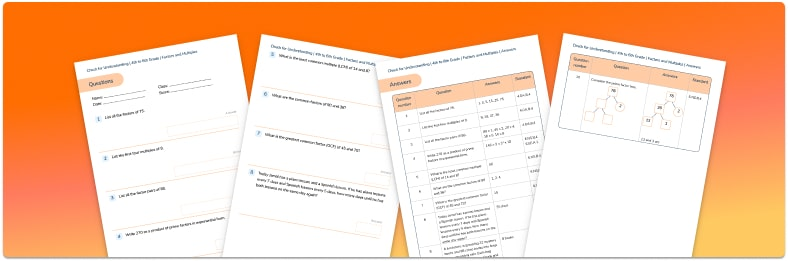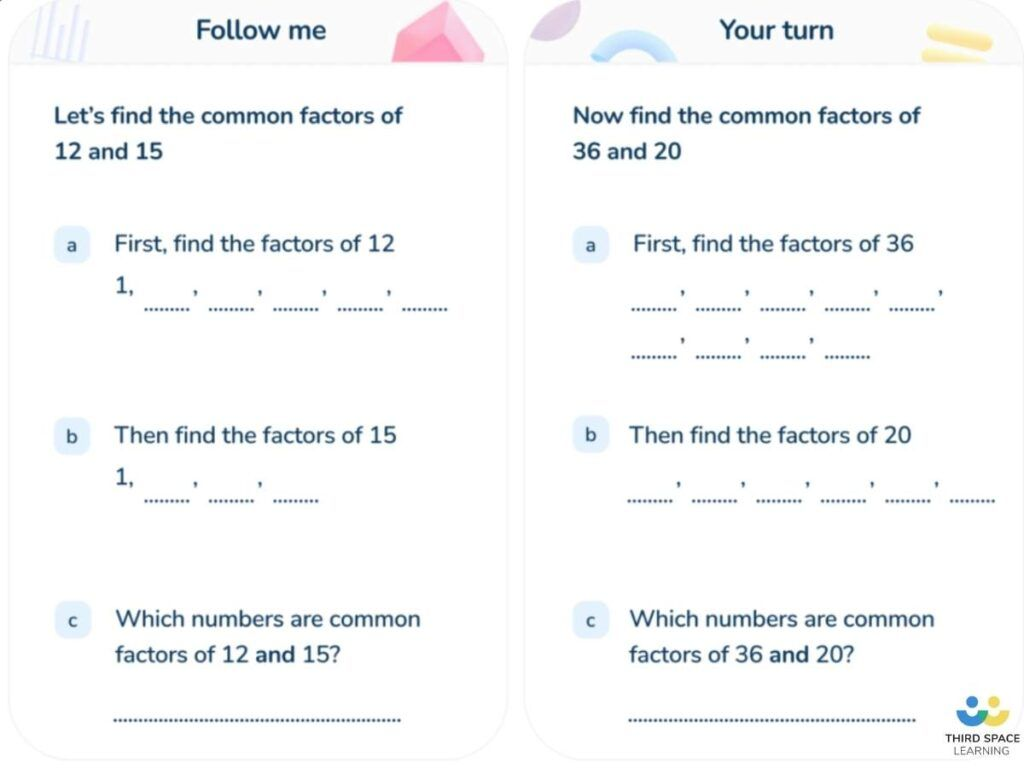Math teaching support you can trustone-on-one tutoring sessionsschools supported

[FREE] Themed Math Problems: Fall Term (Sep – Dec)

5th grade math problems and answers that link to key dates in the Fall Term, including Christmas!

# What Is The Greatest Common Factor: A Guide For Elementary School

In this article, we will be answering the question “what is the greatest common factor” and providing you with all of the information you need to help your students understand this area of math. We’ve also got some questions based around the greatest common factor (GCF) that your students can complete.Factors and Multiples Check for Understanding Quiz

Test your students' knowledge on factors and multiples with this free resource!Download Free Now!

### What is a factor in math?

A factor is a number that fits exactly into another number. For example, 5 is a factor of 10; 7 is a factor of 28. One way of helping children remember factors is to think of factories – factories make things, and factors make up numbers.

Another way is to think of them in pairs (factors are friends – they come in pairs!); for example, 5 x 2 = 10, so 5 and 2 are factors of 10; 7 x 4 = 28, so 7 and 4 are factors of 28. There are 8 factors of 24: 1, 2, 3, 4, 6, 8, 12 and 24 (1 x 24, 2 x 12, 3 x 8 and 4 x 6). Factors are whole numbers rather than decimals.

### What is a common factor?

A common factor is a factor that is shared by multiple numbers. 7 is a common factor of 14 and 21, as it fits exactly into both. Another example would be the common factors of 8 and 12 which are 1, 2 and 4.

A visual way to see common factors is by using factor trees for each of the numbers you are trying to find common factors for. This will allow you to see both the larger numbers and smaller numbers which are common. Another method is writing out each of the factors for the given numbers and underlining any factors which are common.A slide teaching students how to find common factors between numbers from Third Space Learning’s online intervention.

### What is the greatest common factor?

The greatest common factor (GCF) is the largest whole number which is shared by given numbers. For example, common factors of 10 and 20 are 1, 2, 5 and 10, but the highest of those is 10; therefore, the greatest common factor of 10 and 20 is 10.

### How to find the greatest common factor

One quick and easy way of helping children to find the greatest common factor is to ask them to list the factors of each number, underline all the common factors and then find the largest number. For example, the GCF of 18 and 24 is 6:

Another example is that the greatest common factor of 21, 36 and 42 is 3:

### When will my child learn about the greatest common factors?

Factors are introduced in 4th grade. According to the Common Core standards, 4th grade students should be taught to recognize and use factor pairs in the range of 1-100. Students will also learn how to determine if a given whole number is a multiple of a given one-digit number, and to determine whether a given whole number is prime or composite.

This is then extended to common factors in 6th grade. According to the Common Core standards, 6th grade students should learn how to find the greatest common factor of two whole numbers less than or equal to 100, and the least common multiple of two whole numbers less than 12. Students will also use the distributive property to show a sum of two whole numbers 1–100, with a common factor as a multiple of a sum of two whole numbers with no common factor. For example, express 36 + 8 as 4 (9 + 2).

In 5th grade, children are expected to apply their knowledge of common factors to fractions. Students will use the knowledge of common factors to simplify fractions, and finding common factors can be related to finding equivalent fractions.

According to the Texas Essential Knowledge and Skills, students can be expected to apply their knowledge of greatest common factors to finding equivalent fractions and simplifying fractions in the 5th grade.

### How do the greatest common factors relate to other areas of math?

The greatest common factors are useful when simplifying and comparing fractions. If you can work out the greatest common factor of the numerator (top number) and the denominator (bottom number), you can express the fraction in its simplest form.

For example, to simplify 18/24, we divide by the numerator and denominator by the GCF of 18 and 24 (6) to get 3/4.

### Greatest common factor practice questions

1) Calculate the greatest common factor of 24 and 36.

2) Complete this sentence: Every number with a factor of 10 must also have factors of ? and ? and ?.

3) Write all the factors of 30 which are also factors of 20.

4) Use your knowledge of the greatest common factors to express 48/60 in its simplest form.

Wondering about how to explain other key math vocabulary to your students? Check out our Elementary Math Dictionary, or try these elementary math terms:##### Sophie Bartlett
Year 6 Teacher
Sophie teaches mixed age classes at a small school in central England. She is a self confessed grammar pedant and number nerd so we've welcomed her as a regular author and editor for Third Space Learning.
x#### Factors and Multiples Check for Understanding Quiz

Test your students' knowledge on factors and multiples with this free resource!

#### Factors and Multiples Check for Understanding QuizTest your students' knowledge on factors and multiples with this free resource!